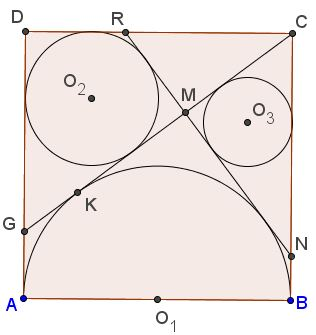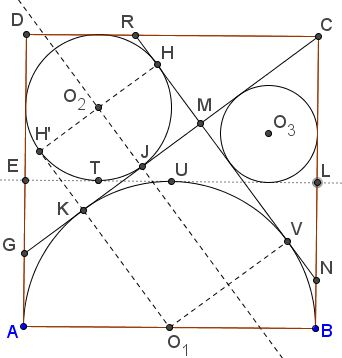# Another Sangaku in Square

This is problem #3.2.5 from Fukagawa & Pedoe's book. It is simple enough, but surprisingly the configuration exhibits several curious properties. In particular, there are several occurrences of the Egyptian 3-4-5 triangle that have been missed on the page specifically devoted to the appearance of that triangle in several sangaku problems. The sangaku is said to have been written in 1838, in the Iwate prefecture, on a tablet which has not survived.

$ABCD$ is a square and the semicircle on $AB$ as diameter, center $O_1,$ lies within it. $CG,$ where $G$ lies on $AD,$ touches this semicircle, and $O_{2}(r_{2})$ is the incircle of $\Delta CDG.$ An external common tangent of the semicircle and $O_{2}(r_{2})$ meets $CD$ in $R,$ $BC$ in $N,$ and intersects $CG$ in $M.$ The circle $O_{3}(r_{3})$ is the incircle of $\Delta CMN.$Prove that $r_{2}:r_{3}=3:2.$

Solution$ABCD$ is a square and the semicircle on $AB$ as diameter, center $O_1,$ lies within it. $CG,$ where $G$ lies on $AD,$ touches this semicircle, and $O_{2}(r_{2})$ is the incircle of $\Delta CDG.$ An external common tangent of the semicircle and $O_{2}(r_{2})$ meets $CD$ in $R,$ $BC$ in $N,$ and intersects $CG$ in $M.$ The circle $O_{3}(r_{3})$ is the incircle of $\Delta CMN.$Prove that $r_{2}:r_{3}=3:2.$

Since the configuration contains several Egyptian triangles, it is worth while to first establish the following

### Lemma

The inradius $r$ of an Egyptian triangle with side lengths $3w,$ $4w,$ $5w,$ equals $w.$

The proof is straightforward. As we know, in a right triangle with legs $a$ and $b$ and hypotenuse $c,$ the inradius equals $(a+b-c)/2.$ Thus, for the Egyptian triangle, we have $r=(3w+4w-5w)/2=w.$Now, $\Delta CDG$ is an Egyptian triangle (this has been established earlier $(\Delta ABH,$ there.) There is a direct derivation. Assume for convenience that the side of the square equals $12.$Let $K$ be the point of tangency of $CG$ and the semicircle. Then $CK=CB=12.$ Let $AG=GK=x.$ In $\Delta CDG,$ $CG^{2}=CD^{2}+DG^{2},$ so that $(12+x)^{2}=12^{2}+(12-x)^{2},$ from which $x=3.$ This exactly means that the sides of $\Delta CDG$ equal $9,$ $12,$ and $15,$ making it an Egyptian triangle. By Lemma, $r_{2}=3.$

Of course, $r_{1}=6.$ Let $EL$ be the midline of square $ABCD$ as shown.Then $EL$ is tangent to both the semicircle and $(O_{2}).$ Let $U$ and $T$ be respective points of tangency. If $J$ the point of tangency of $CG$ and $(O_{2})$ then both $JK$ and $TU$ are internal common tangents of the semicircle and $(O_{2}),$ implying $JK=TU=3.$

Let $KH'$ be a second tangent from $K$ to $(O_{2}).$ Then $H'K=JK$ so that $KJO_{2}H'$ is a rhombus and, since $JK\perp JO_{2},$ it is a square. Thus $H'K\perp KJ$ (i.e., $CG)$ and $K$ lies on $H'O_{1}.$ It follows that $CG\perp H'O_{1}$ and that $H'O_{1}$ is tangent to $(O)_{2})$ at $H'.$

Let $H$ and $V$ on $NR$ be the points of tangency of $NR$ with $(O_{2})$ and $(O_{1}),$ respectively. $HV$ is parallel (and equal $H'O_{1})$ and so $HV\perp CG.$ In other words, $CG\perp RN.$ Thus triangles $CNR,$ $CMR,$ and $CMN$ are all similar to $\Delta CDG$ and, hence, are all Egyptian. As the final step we need to evaluate the "size" of $\Delta CMN.$

We have already found that $GK=AG=3.$ Also $JK=JM=3.$ Thus $GM=9,$ whereas $CG=15,$ giving $CM=6.$ From here the inradius $r_2$ of $\Delta CMN$ equals $2,$ and this solves the problem.

### Extra

The configuration has several additional properties:

1. Let $F$ be the intersection of $EL$ and $NR.$ Then $C,O_{3},F,O_{1}$ are collinear. $FL=\frac{1}{4}EL.$2. $FO_{1}GO_{2}$ is a square.

3. $CO_{1}O_{2}$ is a right isosceles triangle.

4. $G, O_{2},R$ are collinear and $DR=\frac{3}{8}CD.$

5. $S$ the intersection of $O_{2}F$ with $CG$ is the midpoint of $O_{2}F$ and $CG.$

6. $D,M,L$ are collinear.

7. $BN=\frac{1}{6}BC.$

### References

1. H. Fukagawa, D. Pedoe, Japanese Temple Geometry Problems, The Charles Babbage Research Center, Winnipeg, 1989

Write to:

Charles Babbage Research Center
P.O. Box 272, St. Norbert Postal Station
Winnipeg, MB